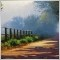# Confusion with this piece of code[Deleted]

I am sure there is a simple explanation but I would be grateful to anyone who can explain this to me. In the following snippet of code e1 is not initialised with any value simply set up as "double e1". If this is the case how can this calculation work since e1 is dependent on a value of e1 already in that register? This is within an indicator.

//---- indicator calculation
for(int i = Bars - 1; i >= 0; i--)
{
cci[i] = iCCI(NULL, 0, CCI_Period, PRICE_TYPICAL, i);
e1 = w1*cci[i] + w2*e1;
e2 = w1*e1 + w2*e2;
e3 = w1*e2 + w2*e3;
e4 = w1*e3 + w2*e4;
e5 = w1*e4 + w2*e5;
e6 = w1*e5 + w2*e6;
cci[i] = c1*e6 + c2*e5 + c3*e4 + c4*e3;
//----116

Acerinvest wrote >>

I am sure there is a simple explanation but I would be grateful to anyone who can explain this to me. In the following snippet of code e1 is not initialised with any value simply set up as "double e1". If this is the case how can this calculation work since e1 is dependent on a value of e1 already in that register? This is within an indicator.

//---- indicator calculation
for(int i = Bars - 1; i >= 0; i--)
{
cci[i] = iCCI(NULL, 0, CCI_Period, PRICE_TYPICAL, i);
e1 = w1*cci[i] + w2*e1;
e2 = w1*e1 + w2*e2;
e3 = w1*e2 + w2*e3;
e4 = w1*e3 + w2*e4;
e5 = w1*e4 + w2*e5;
e6 = w1*e5 + w2*e6;
cci[i] = c1*e6 + c2*e5 + c3*e4 + c4*e3;
//----

if the double varialble is not assigned an initial value,it will get zero value.

you may try :

int init()

{

.............

..............

Print("e1=",e1,",e2=",e2,",e3=",e3);
//----
return(0); }

to see the output of print function,you will find the reuslt.476

In that indy, after a few cycle the meaningless value will fade to the significant ones.[Deleted]

Thanks. That helps a lot. If I am picking up on the final closed bar in the array is that in register 1 or in register bars -1 ? Sorry if I am being a bit dense on this.476

Acerinvest:

Thanks. That helps a lot. If I am picking up on the final closed bar in the array is that in register 1 or in register bars -1 ? Sorry if I am being a bit dense on this.

In general cases : the 'final closed bar' / 'latest completed bar' of series arrays & Indicators calculated using preset Index buffer(s) is always bar index 1. Applied whether you used an incremental or decremental loop. However regular arrays that are using iMAOnArray will have the 'normal' order (i.e. latest bar value is indexed Bars-1) if ArraySetAsSeries is not used or not set to True. Since the default calculation for iMAOnArray is normal order (as opposed to Series/reversed order- latest bar indexed 0). Others feel free to correct me if this is inaccurate.